### 1. Introduction of Body Density data.

This is a comprehensive dataset that lists estimates of the percentage of body fat determined by underwater weighing and various body circumference measurements for 252 men. These data were generously supplied by Dr. A. Garth Fisher who gave permission to freely distribute the data and use for non-commercial purposes. These data are used to produce the predictive equations for lean body weight given in the abstract Generalized body composition prediction equation for men using simple measurement techniques, K.W. Penrose, A.G. Nelson, A.G. Fisher, FACSM, Human Performance Research Center, Brigham Young University, Provo, Utah 84602 as listed in Medicine and Science in Sports and Exercise, vol. 17, no. 2, April 1985, p. 189.(http://wiki.stat.ucla.edu/socr/index.php/SOCR_Data_BMI_Regression#References)

### 2. Theories about BMI and Body Fat Percentage

Some experts tout BMI(body mass index) as the most accurate and simple way to determine the effect of weight on your health. In fact, most recent medical research uses BMI as an indicator of someone’s health status and disease risk. Some debate about which values on the BMI scale the thresholds for ‘underweight’, ‘overweight’ and ‘obese’ should be set. However, the followings are used for the criteria.(http://healthiack.com/body-fat-percentage-calculator)
BMI < 18.5 : underweight,
18.5 < BMI < 25 : optimal weight,
25 < BMI < 30 : overweight,
BMI > 30 : obese.

Meanwhile, in September 2000, the American Journal of Clinical Nutrition published a study showing that body-fat percentage may be a better measure of your risk of weight-related diseases than BMI.(http://www.webmd.com/diet/body-fat-measurement). Percentage of body fat for an individual can be estimated by the Siri’s formula(1956) once body density has been determined. The American Council on Exercise provides the following ranges for men’s body-fat percentage:

essential fat : 2-5%
athletes : 6-13%
fitness : 14-17%
Normal : 18-24%
obese : more than 24%

Which one is a better and more accurate measure of body fat? This question remains to be studied further. Instead, the main goal of this analysis is to find out the relationships between ‘Body Fat Percentage’ and other predictors as well as ‘Body Mass Index’ and others.

### 3. The variables of Body Density data.

The body density dataset includes the following 15 variables listed from left to right:
Density : Density determined from underwater weighing
Fat : Percent body fat from Siri’s (1956) equation
Age : Age (years)
Weight : Weight (kg)
Height : Height (cm)
Neck : Neck circumference (cm)
Chest: Chest circumference (cm)
Abdomen : Abdomen circumference (cm)
Hip : Hip circumference (cm)
Thigh : Thigh circumference (cm)
Knee : Knee circumference (cm)
Ankle : Ankle circumference (cm)
Biceps : Biceps (extended) circumference (cm)
Forearm : Forearm circumference (cm)
Wrist : Wrist circumference (cm)

New variable MassIndex will be created as the ratio of Weight(kg) to Height(m) squared for the measure of Body Mass Index in addition to the above variables. Model Selection process and regressions for both response variables, Fat and MassIndex, will be compared to explain which measure explains this data better.

### 4.Reading Body Density Data and Creating a new dataset

library(car)
library(stargazer)
library(Zelig)
bmi <- read.table("K:/QC/Soc/SOC712/Homework/BMI.txt",header=TRUE)
summary(bmi)
##     Density           Fat             Age            Height
##  Min.   :0.995   Min.   : 0.00   Min.   :22.00   Min.   : 74.93
##  1st Qu.:1.041   1st Qu.:12.47   1st Qu.:35.75   1st Qu.:173.35
##  Median :1.055   Median :19.20   Median :43.00   Median :177.80
##  Mean   :1.056   Mean   :19.15   Mean   :44.88   Mean   :178.18
##  3rd Qu.:1.070   3rd Qu.:25.30   3rd Qu.:54.00   3rd Qu.:183.51
##  Max.   :1.109   Max.   :47.50   Max.   :81.00   Max.   :197.49
##      Weight            Neck           Chest           Abdomen
##  Min.   : 53.75   Min.   :31.10   Min.   : 79.30   Min.   : 69.40
##  1st Qu.: 72.12   1st Qu.:36.40   1st Qu.: 94.35   1st Qu.: 84.58
##  Median : 80.06   Median :38.00   Median : 99.65   Median : 90.95
##  Mean   : 81.16   Mean   :37.99   Mean   :100.82   Mean   : 92.56
##  3rd Qu.: 89.36   3rd Qu.:39.42   3rd Qu.:105.38   3rd Qu.: 99.33
##  Max.   :164.72   Max.   :51.20   Max.   :136.20   Max.   :148.10
##       Hip            Thigh            Knee           Ankle
##  Min.   : 85.0   Min.   :47.20   Min.   :33.00   Min.   :19.1
##  1st Qu.: 95.5   1st Qu.:56.00   1st Qu.:36.98   1st Qu.:22.0
##  Median : 99.3   Median :59.00   Median :38.50   Median :22.8
##  Mean   : 99.9   Mean   :59.41   Mean   :38.59   Mean   :23.1
##  3rd Qu.:103.5   3rd Qu.:62.35   3rd Qu.:39.92   3rd Qu.:24.0
##  Max.   :147.7   Max.   :87.30   Max.   :49.10   Max.   :33.9
##      Biceps         Forearm          Wrist
##  Min.   :24.80   Min.   :21.00   Min.   :15.80
##  1st Qu.:30.20   1st Qu.:27.30   1st Qu.:17.60
##  Median :32.05   Median :28.70   Median :18.30
##  Mean   :32.27   Mean   :28.66   Mean   :18.23
##  3rd Qu.:34.33   3rd Qu.:30.00   3rd Qu.:18.80
##  Max.   :45.00   Max.   :34.90   Max.   :21.40

The above table shows the Body Density Dataset having 15 variables and 252 observations.
Next step is to create new variable MassIndex using mutate function in dplyr package. As a result, 16 variables are in the data named bmi2.

library(dplyr)
bmi2 <- mutate(bmi, MassIndex = Weight/(Height/100)^2)
names(bmi2)
##   "Density"   "Fat"       "Age"       "Height"    "Weight"
##   "Neck"      "Chest"     "Abdomen"   "Hip"       "Thigh"
##  "Knee"      "Ankle"     "Biceps"    "Forearm"   "Wrist"
##  "MassIndex"

### 5. Comparing the distributions of Body Mass Index and Body Fat Percent using categorization.

To compare the distributions of 2 response variables
the following criteria are used to categorize the types of weights for Body Mass Index.

Underweight Less than 18.5
Recommended 18.6 to 24.9
Overweight 25.0 to 29.9
Obese 30 or greater

attach(bmi2)
bmi2$WeightGroup[MassIndex < 18.5] <- 1 bmi2$WeightGroup[MassIndex >= 18.5 & MassIndex <25] <- 2
bmi2$WeightGroup[MassIndex >= 25 & MassIndex < 30] <- 3 bmi2$WeightGroup[MassIndex >= 30 ] <-4
bmi2$WeightGroup[is.na(MassIndex)] <- NA detach(bmi2) WeightType <- c("Underweight", "Normalweight", "Overweight", "Obese") bmi2$WeightGroup <-factor(bmi2$WeightGroup, labels = WeightType) Now the variable WeightGroup has 4 categories for the types of weight based on the Body Mass Index. Then the following criteria are used to categorize the types of weights for Body Fat Percent. essential fat : 2-5% athletes : 6-13% fitness : 14-17% Normal : 18-24% obese : more than 24% attach(bmi2) bmi2$WeightGroup2[Fat < 6] <- 1
bmi2$WeightGroup2[Fat >= 6 & Fat < 14] <- 2 bmi2$WeightGroup2[Fat >= 14 & Fat < 18] <- 3
bmi2$WeightGroup2[Fat >= 18 & Fat < 25 ] <- 4 bmi2$WeightGroup2[Fat >= 25] <- 5
bmi2$WeightGroup2[is.na(Fat)] <- NA detach(bmi2) WeightType2 <- c("EssentialFat", "Athletes", "Fitness", "Average", "Obese") bmi2$WeightGroup2 <-factor(bmi2$WeightGroup2, labels = WeightType2) Now the variable WeightGroup2 has 5 categories for the types of weight based on the Boyd Fat Percent. The following results shows the number of male participants categorized as underweight, normal weight, overweight, and obese according to their Body Mass Index Value. And they are also categorized as underweight, normal weight, overweight, and obese with their Body Fat Percent. summary(bmi2$WeightGroup)
##  Underweight Normalweight   Overweight        Obese
##            1          124          102           25
summary(bmi2\$WeightGroup2)
## EssentialFat     Athletes      Fitness      Average        Obese
##           12           63           37           74           66

As we can see with the above comparison, Body Mass Index and Body Fat Percent have quite different distributions especially for both tails. Body Fat Percent has much thicker tails on both sides(for EssentialFat and Obese) than Body Mass Index.

### 6. Comparing Regression models for Body Mass Index and Body Fat Percent

#### 1) Selection of the significant predictors using general linear model

To select significant predictors for the response variable ‘Fat’, linear regression is performed.
The below table shows that only ‘Density’ has a significant linear relationship with ‘Fat’.

summary(bmi.mod1 <- lm(Fat ~ Density + Age + Height + Weight + Neck + Chest + Abdomen + Hip + Thigh + Knee + Ankle + Biceps + Forearm + Wrist, data = bmi2))
##
## Call:
## lm(formula = Fat ~ Density + Age + Height + Weight + Neck + Chest +
##     Abdomen + Hip + Thigh + Knee + Ankle + Biceps + Forearm +
##     Wrist, data = bmi2)
##
## Residuals:
##     Min      1Q  Median      3Q     Max
## -8.4357 -0.3724 -0.1275  0.2156 15.1474
##
## Coefficients:
##               Estimate Std. Error t value Pr(>|t|)
## (Intercept)  4.500e+02  1.071e+01  42.005   <2e-16 ***
## Density     -4.112e+02  8.258e+00 -49.796   <2e-16 ***
## Age          1.259e-02  9.626e-03   1.308    0.192
## Height      -3.142e-03  1.120e-02  -0.281    0.779
## Weight       2.217e-02  3.520e-02   0.630    0.529
## Neck        -2.846e-02  6.938e-02  -0.410    0.682
## Chest        2.678e-02  2.936e-02   0.912    0.363
## Abdomen      1.857e-02  3.175e-02   0.585    0.559
## Hip          1.917e-02  4.343e-02   0.441    0.659
## Thigh       -1.676e-02  4.303e-02  -0.389    0.697
## Knee        -4.639e-03  7.162e-02  -0.065    0.948
## Ankle       -8.568e-02  6.576e-02  -1.303    0.194
## Biceps      -5.505e-02  5.087e-02  -1.082    0.280
## Forearm      3.386e-02  5.953e-02   0.569    0.570
## Wrist        7.345e-03  1.617e-01   0.045    0.964
## ---
## Signif. codes:  0 '***' 0.001 '**' 0.01 '*' 0.05 '.' 0.1 ' ' 1
##
## Residual standard error: 1.274 on 237 degrees of freedom
## Multiple R-squared:  0.9781, Adjusted R-squared:  0.9768
## F-statistic: 756.3 on 14 and 237 DF,  p-value: < 2.2e-16

Again, linear regression is performed to select significant predictors for the response variable ‘MassIndex’. As a result, the predictors; Height, Weight,Neck, Chest, Abdomen, Thigh, and Knee are chosen for their significance.

summary(bmi.mod2 <- lm(MassIndex ~ Density + Age + Height + Weight + Neck + Chest + Abdomen + Hip + Thigh + Knee + Ankle + Biceps + Forearm + Wrist, data = bmi2))
##
## Call:
## lm(formula = MassIndex ~ Density + Age + Height + Weight + Neck +
##     Chest + Abdomen + Hip + Thigh + Knee + Ankle + Biceps + Forearm +
##     Wrist, data = bmi2)
##
## Residuals:
##      Min       1Q   Median       3Q      Max
## -16.3523  -2.0685  -0.3381   1.6979  28.2505
##
## Coefficients:
##               Estimate Std. Error t value Pr(>|t|)
## (Intercept) 229.605482  30.330244   7.570 8.26e-13 ***
## Density      -6.343269  23.380600  -0.271 0.786393
## Age          -0.007158   0.027253  -0.263 0.793040
## Height       -1.029726   0.031703 -32.480  < 2e-16 ***
## Weight        1.154493   0.099651  11.585  < 2e-16 ***
## Neck         -0.515661   0.196416  -2.625 0.009219 **
## Chest        -0.344475   0.083132  -4.144 4.76e-05 ***
## Abdomen      -0.330356   0.089894  -3.675 0.000294 ***
## Hip          -0.146461   0.122946  -1.191 0.234743
## Thigh        -0.325452   0.121819  -2.672 0.008072 **
## Knee          0.654171   0.202773   3.226 0.001432 **
## Ankle        -0.246928   0.186169  -1.326 0.185996
## Biceps       -0.272801   0.144028  -1.894 0.059431 .
## Forearm       0.100265   0.168550   0.595 0.552497
## Wrist        -0.088536   0.457726  -0.193 0.846792
## ---
## Signif. codes:  0 '***' 0.001 '**' 0.01 '*' 0.05 '.' 0.1 ' ' 1
##
## Residual standard error: 3.608 on 237 degrees of freedom
## Multiple R-squared:  0.8655, Adjusted R-squared:  0.8575
## F-statistic: 108.9 on 14 and 237 DF,  p-value: < 2.2e-16

#### 2) Model Selection using the Akaike information criterion (AIC)

In generalized linear regression models, the optimzed model can be obtained by selecting active predictors with Akaike information criterion(AIC) or Bayesan information criterion(BIC). Step function with backward method is used to select variables for the optimized subset models by the Akaike information criterion (AIC) for the given set of data.

(bmi.backward1 <- step(bmi.mod1, scope = list(lower ~ Density), trace=0))
##
## Call:
## lm(formula = Fat ~ Density + Age + Chest, data = bmi2)
##
## Coefficients:
## (Intercept)      Density          Age        Chest
##   452.23095   -415.90774      0.01289      0.05319

As a result, Density, age, and chest are selected in the optimized model for the response variable Fat.

Then the fitted values for bmi.mod1 with all the regressors and the fitted values for subset models selected by backward step function are compared in the below graph.

plot(fitted(bmi.mod1) ~ fitted(bmi.backward1))
abline(0,1)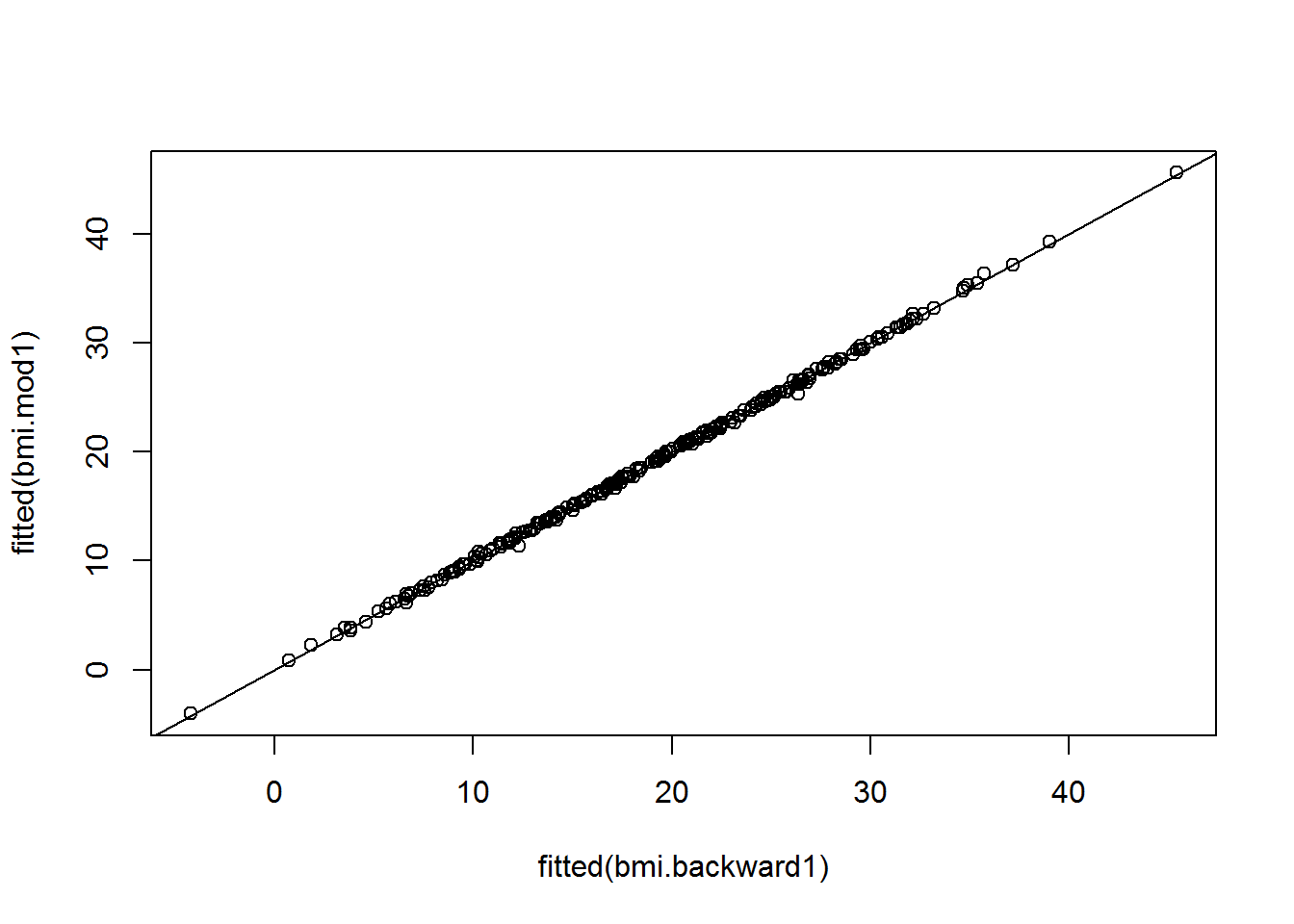cor(fitted(bmi.mod1), fitted(bmi.backward1))
##  0.9997243

The change in the fitted values is relatively small, and the two sets of fitted values have correlation .99. So we conclude that the subset model and the full model provide essentially the same information about the value of the response given predicors.(Fox and Weisberg 2011)

Similary, Step function with backward method is used to select variables for the optimized subset models for the response variable MassIndex for the given set of data.

(bmi.backward2 <- step(bmi.mod2, scope = list(lower ~ Density), trace=0))
##
## Call:
## lm(formula = MassIndex ~ Height + Weight + Neck + Chest + Abdomen +
##     Thigh + Knee + Biceps, data = bmi2)
##
## Coefficients:
## (Intercept)       Height       Weight         Neck        Chest
##    207.7371      -1.0187       1.0607      -0.4758      -0.3171
##     Abdomen        Thigh         Knee       Biceps
##     -0.3352      -0.3480       0.5859      -0.2238

Then the fit of the full and subset models is compared by looking at the corresponding fitted values.

plot(fitted(bmi.mod2) ~ fitted(bmi.backward2))
abline(0,1)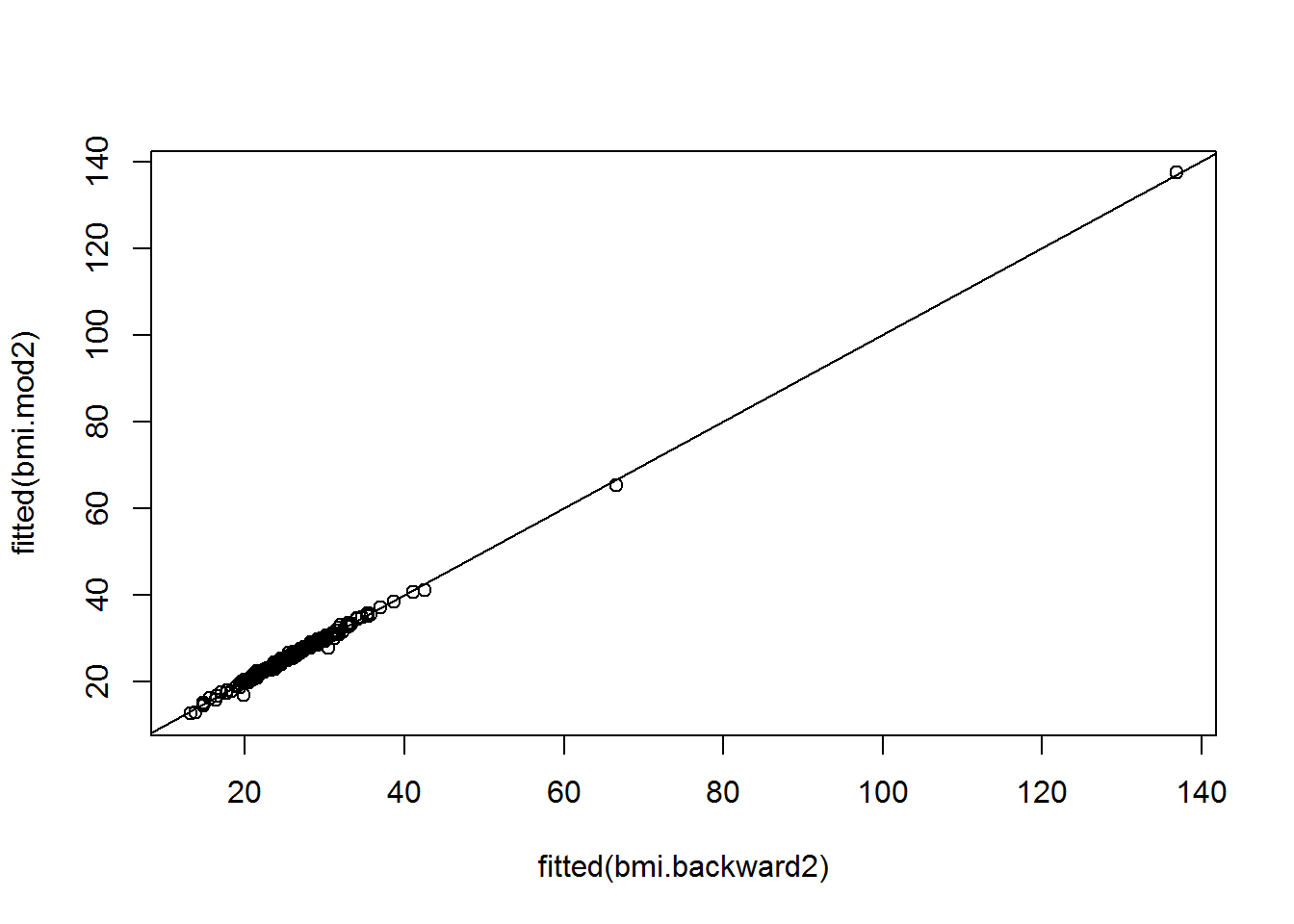cor(fitted(bmi.mod2), fitted(bmi.backward2))
##  0.9986755

Again the subset model and the full model provide essentially the same information abouth the value of the response given predictors.

#### 3) Regression outputs with 2 subsets.

Based on the above results, 2 different subsets of data are selected. First subset ‘subbmi1’ has Fat(as response variable) and density after removing all other variables. Second subset ‘subbmi2’ has variables: MassIndex(as response variable) and Height, Weight,Neck, Chest, Abdomen, Thigh, and Knee as predictors.

subbmi1 <- select(bmi2, Fat, Density)
names(subbmi1)
##  "Fat"     "Density"
subbmi2 <- select(bmi2, MassIndex, Height, Weight,Neck, Chest, Abdomen, Thigh, Knee)
names(subbmi2)
##  "MassIndex" "Height"    "Weight"    "Neck"      "Chest"     "Abdomen"
##  "Thigh"     "Knee"

Then regressions results for 2 different subsets and response variables are compared using stargazer package.(Hlavac 2014) The table shows that FAT~Density regression model has higher R-sqaure value than MassIndex regression model. Multiple R-squared value is 0.976 which implies that approximately 97.6 % of the vairiability of the dependant variable is explained by the fitted regression line. MassIndex regression has 86.2% R-squre. The weighted combination of the 7 predictor variables explained approximately 86.2% of the variance of the dependent variable in the MassIndex regression.
Density=-434.360 implies that Fat will be expected to decrease by 434.360 percent for an additonal density unit. Another resonse variable MassIndex has negative linear relationship with Height, Neck, Chest, Abdomen, and Thigh but postive linear relationship with Weight and Knee.

library(stargazer)

bmi.m1 <- lm(Fat ~ Density, data =subbmi1)

bmi.m2 <- lm(MassIndex ~ Height+Weight+Neck+Chest+ Abdomen+Thigh+Knee, data =subbmi2)

stargazer(bmi.m1, bmi.m2, title="Comparison of 2 Regression outputs",type="html", single.row=TRUE)
 Dependent variable: Fat MassIndex (1) (2) Density -434.360*** (4.334) Height -1.017*** (0.030) Weight 1.040*** (0.075) Neck -0.537*** (0.172) Chest -0.337*** (0.080) Abdomen -0.317*** (0.060) Thigh -0.391*** (0.098) Knee 0.597*** (0.189) Constant 477.650*** (4.576) 206.708*** (10.790) Observations 252 252 R2 0.976 0.862 Adjusted R2 0.976 0.858 Residual Std. Error 1.307 (df = 250) 3.606 (df = 244) F Statistic 10,044.030*** (df = 1; 250) 217.073*** (df = 7; 244) Note: p<0.1; p<0.05; p<0.01

### 7. Unusual Data Check and removal

#### 1) Outlier check

Regression outliers are y values that are unusual conditional on the values of the predictors. The Quantile-quantile plot and the outlierTest function for the regression model (Fat ~ Density) shows that observations 96 and 48 are outliers.

qqPlot(bmi.m1, id.n=4)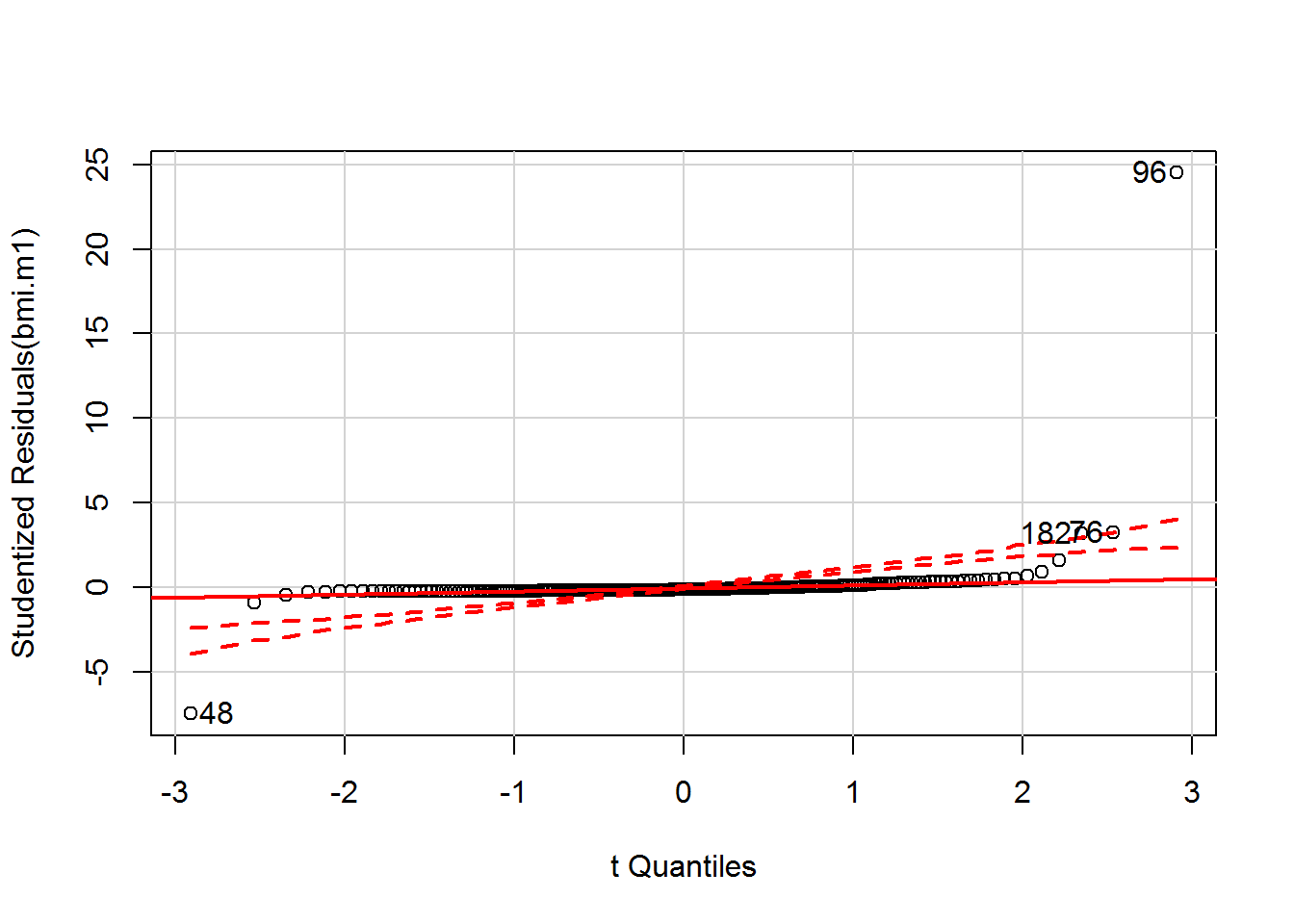##  48 182  76  96
##   1 250 251 252
outlierTest(bmi.m1)
##     rstudent unadjusted p-value Bonferonni p
## 96 24.505612         2.6448e-68   6.6650e-66
## 48 -7.457113         1.4662e-12   3.6949e-10

The Quantile-quantile plot and the outlierTest function for the regression model (MassIndex ~ Height+Weight+Neck+Chest+ Abdomen+Thigh+Knee) shows that observations 42 and 39 are outliers.

qqPlot(bmi.m2, id.n=4)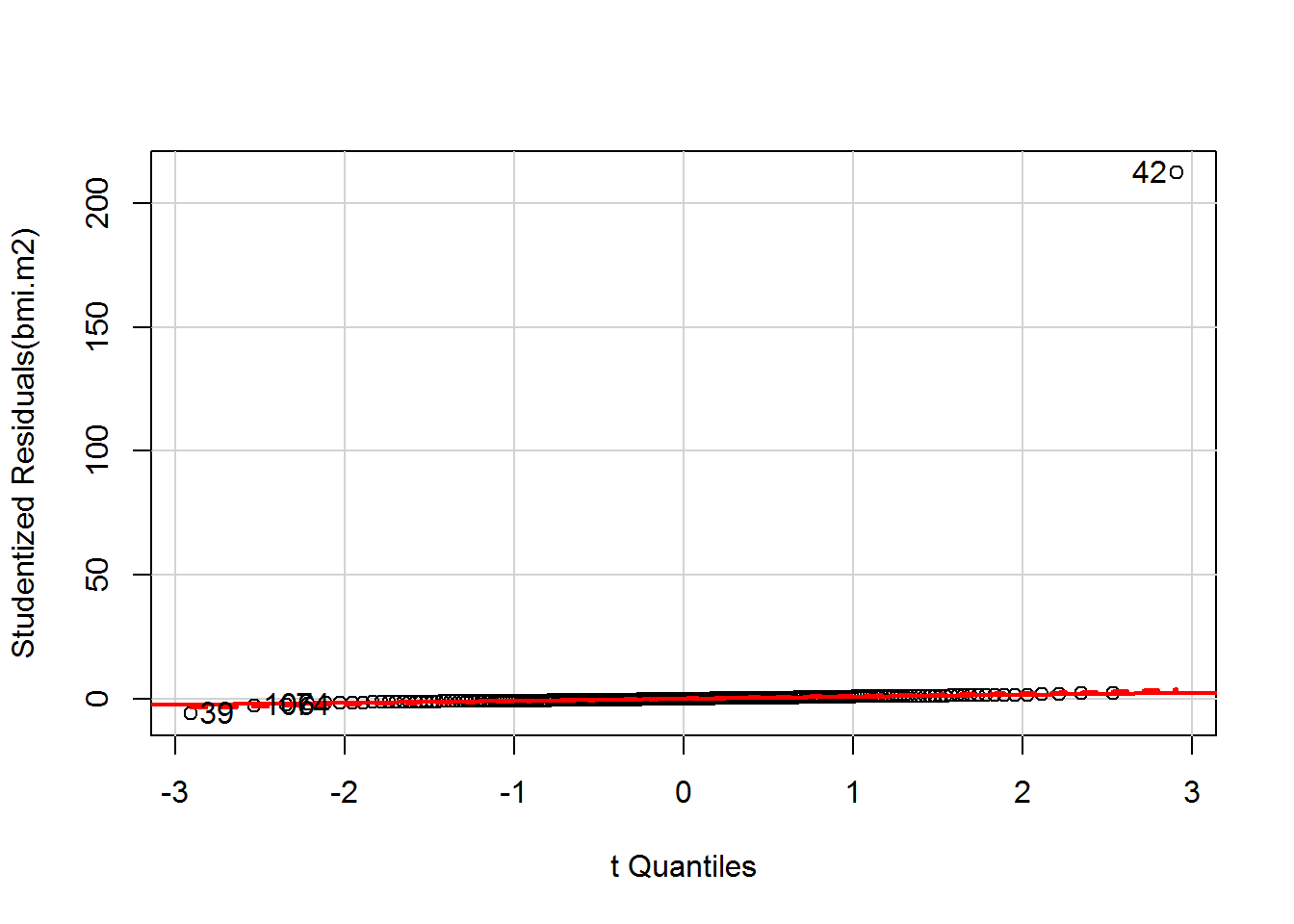##  39 106  74  42
##   1   2   3 252
outlierTest(bmi.m2)
##      rstudent unadjusted p-value Bonferonni p
## 42 212.253315        7.1043e-278  1.7903e-275
## 39  -5.977953         8.0100e-09   2.0185e-06

#### 2) Leverage Check

Observations with high leverage are relatively far from the center of the regressor space, and have potentially greater influence on the least-squares regression coefficients. The function influenceIndexPlot in car package shows hat values and Cook’s distances, the most common measures of leverage.(Fox and Weisberg 2011) As a result, observations 96, 48, 182, 36 and 216 have high leverage for the response variable Fat. Among them 96, 182, and 216 are flagged in both graphs.

influenceIndexPlot(bmi.m1, vars=c("Cook", "hat"),  id.n=4)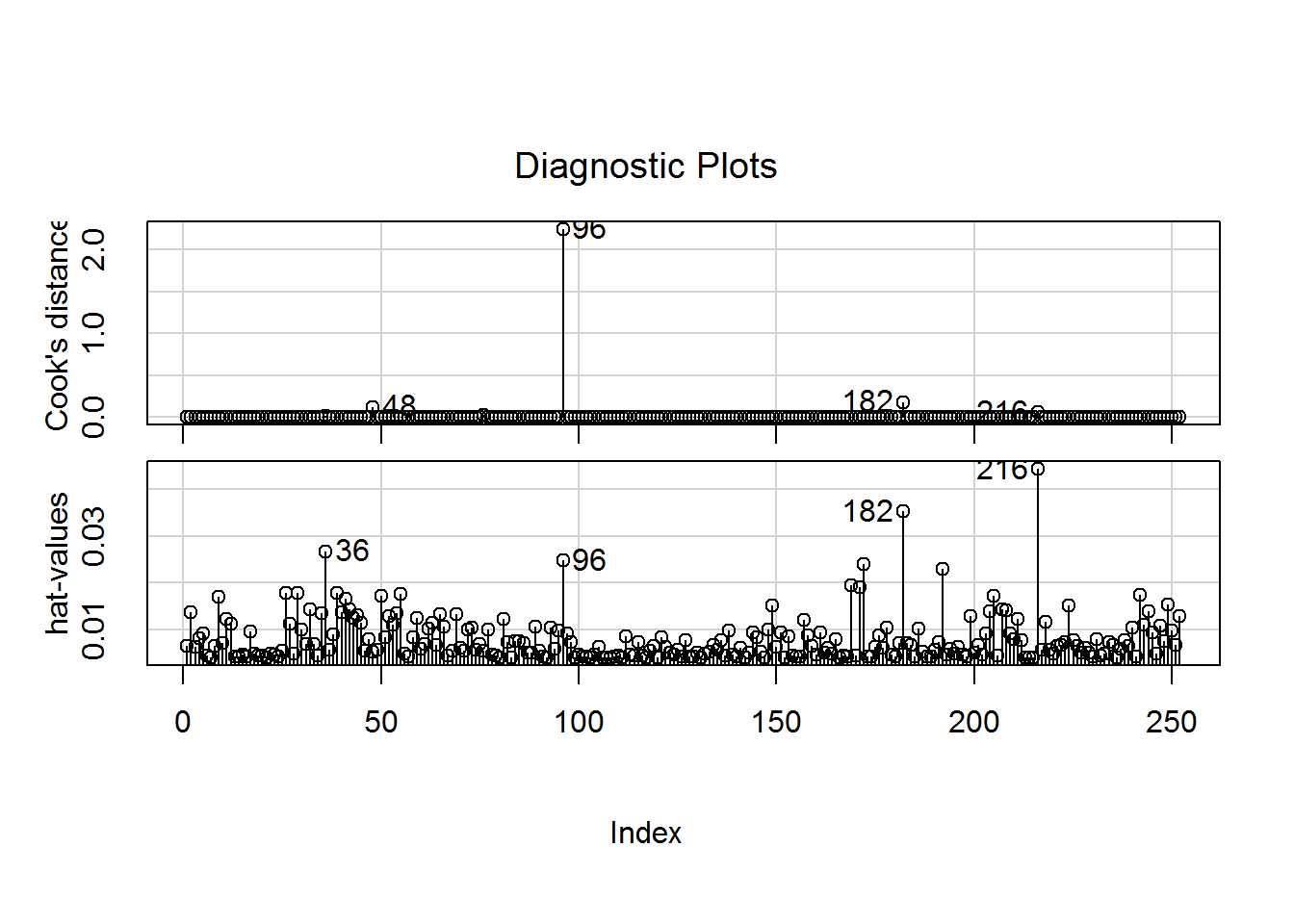So the following results shows that Observations 42, 39 have high leverage for the response variable MassIndex.

influenceIndexPlot(bmi.m2, vars=c("Cook", "hat"), id.n=4)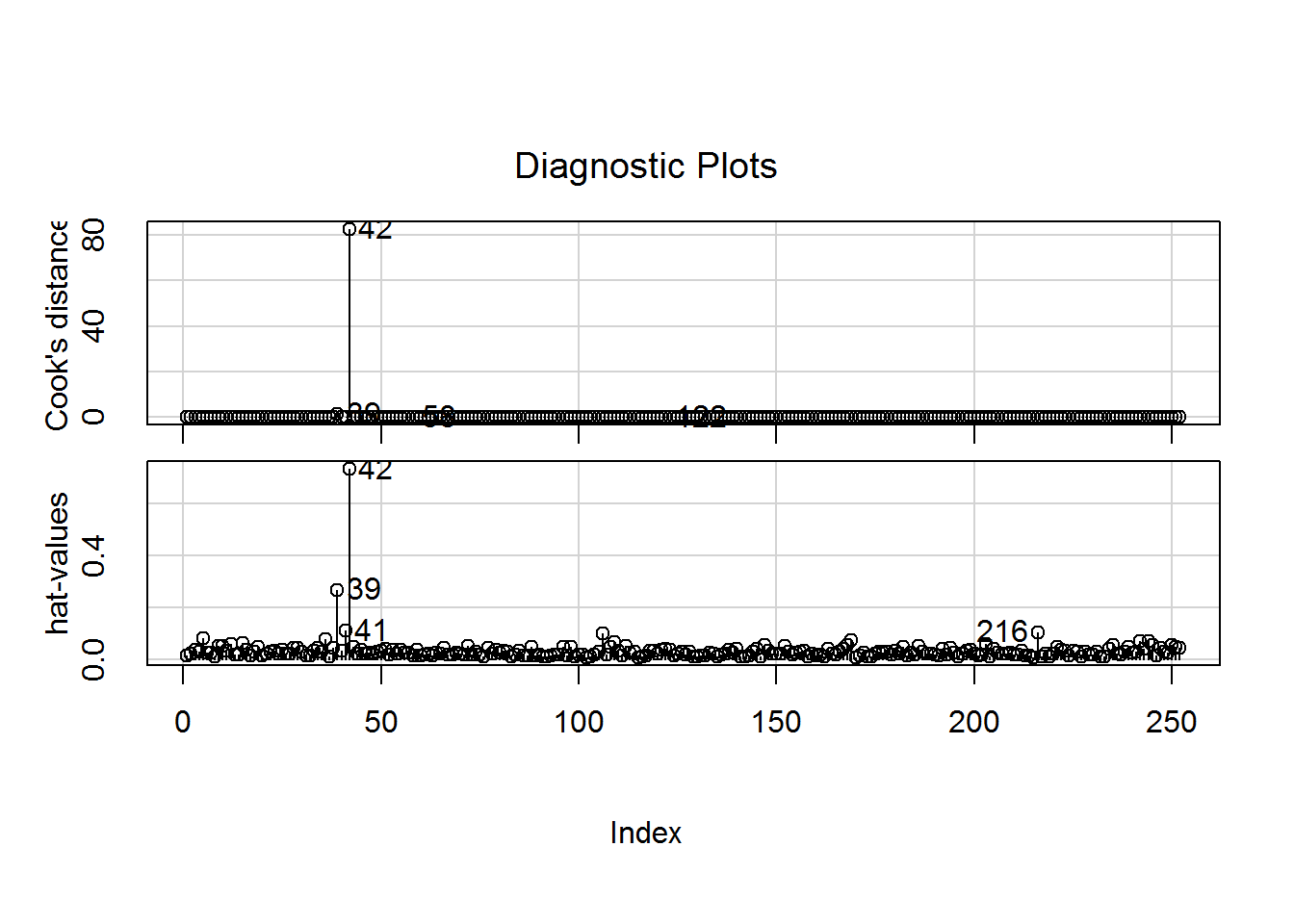According to the above diagnostics, the outliers and high leverage observations are removed. 4 observations are removed from the regression model for the response variable Fat and 2 observations are removed from the regression model fro the response variable MassIndex.

bmi.m3 <- update(bmi.m1, subset = -c(96, 48, 182, 216))

bmi.m4 <- update(bmi.m2, subset = -c(42, 39))

#### 3) Comparing Regression Outputs without Unusual Data

Linear regression analysis are performed again for 2 subsets without influential values. Then the results are compared in the table below.

library(stargazer)

stargazer(bmi.m3, bmi.m4, title="Comparison of 2 Regression outputs without unusual data",type="html", single.row=TRUE)
 Dependent variable: Fat MassIndex (1) (2) Density -443.388*** (1.161) Height -0.277*** (0.004) Weight 0.302*** (0.007) Neck -0.008 (0.013) Chest 0.009 (0.006) Abdomen 0.013*** (0.005) Thigh 0.006 (0.007) Knee -0.027* (0.014) Constant 487.120*** (1.225) 49.141*** (1.158) Observations 248 250 R2 0.998 0.994 Adjusted R2 0.998 0.994 Residual Std. Error 0.333 (df = 246) 0.258 (df = 242) F Statistic 145,970.800*** (df = 1; 246) 5,955.936*** (df = 7; 242) Note: p<0.1; p<0.05; p<0.01

Without outliers and high leverage observations 2 regression models show significantly improved outputs for the dataset. Especially regression outcome for the response variable(MassIndex) has much higher R square values than the previous regression model. This change can be explained by the effect of outliers and high leverage values in the dataset.

### 8. Discussion of the results

Both Body Mass Index (BMI) and Body Fat Percent are measures of the body fat. For the Body Density Data 2 different response variables are used to identify the relationsip between each response variable and their own significant predictors to determine which body fat measure represents this dataset better. The result of this analysis shows that Body Fat percent(Fat) has a significant negative linear relationship only with the variable Denstiy since the fomula for Body Fat Percent is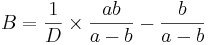and Body Density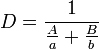, where
D = Body Density (gm/cm3)
A = proportion of lean body tissue
B = proportion of fat tissue (A+B=1)
a = density of lean body tissue (gm/cm3)
b = density of fat tissue (gm/cm3)

Meanwhile, Body Mass Index(MassIndex) has strong linear relationships with multiple predictors since Body Mass Index can be calculated by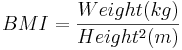and Height and Weight are also correlated with other measurements such as Abdomen, Thigh, etc.

Accurate measurement of body fat is inconvenient or costly and it is desirable to have easy methods of estimating body fat that are cost-effective and convenient. However, most of the body measures except for the Body Fat Percent and the Body Density are relatively easier to obtain for data collection. Body Mass Index is also calculated simply from weight and height of an individual.
Conclusively, Body Mass Index is a more conveniet and less costly method for Body fat measures since it is strongly correlated with most body measures except Body Fat Percent and Density. One plausible problem with Body Mass Index(‘MassIndex’) regression is that there is a possiblity of multicollinearity among predictor variables since all body measures are highly correlated.

However, What is the better or more accurate measure of human body fat remains to be answered with further medical reseaches.

### References

Fox, John, and Harvey Sanford Weisberg. 2011. An R Companion to Applied Regression. 2nd ed. Thousand Oaks, CA: Sage Publications.

Hlavac, Marek(2014). 2014. Stargazer:LaTex Code and ASCII Text for Well-Formatted Regression and Summary Staistics Tables. http://CRAN.R-project.org/package=stargazer.